# Gamma Ornstein-Uhlenbeck Stochastic Clocks

In this post, we consider one particular specification of the background driving Lévy process in the general Ornstein-Uhlenbeck stochastic clock dynamics introduced in a previous post. We show that a compound Poisson process with exponentially distributed increments yields a gamma stationary distribution for the instantaneous rate of activity. We also discuss how the problem could be approached from the other end by imposing the stationary distribution and finding the corresponding background driving Lévy process.

Related Posts

Construction via the Background Driving Lévy Process

Let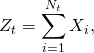where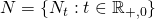is a Poisson process with arrival rate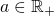and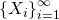is a sequence of independent identically distributed (i.i.d.) exponential random variables with mean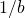for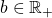. See Barndorff-Nielsen and Shephard (2001a) or Chapter 5.2 in Schoutens (2003) for this construction of the gamma Ornstein-Uhlenbeck process. The characteristic function of the background driving Lévy process is given bywhereis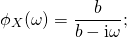see e.g. Proposition 3.4 in Cont and Tankov (2004). The corresponding characteristic exponent is thus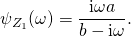The characteristic function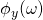of the instantaneous rate of activity is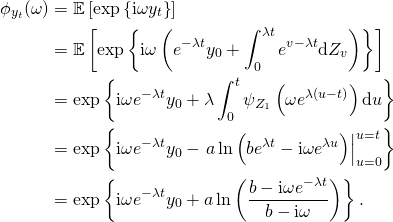Here, we used the previously obtained result that links the expected value of an exponential stochastic integral with respect to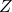to the exponential integral over the characteristic exponent, i.e.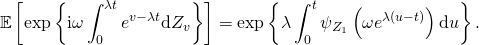Taking the limit yieldsWe recognize this as the characteristic function of a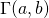gamma distributed random variable as previously claimed.

Construction via the Stationary Distribution

Without assuming a particular form of the background driving Lévy process, we start from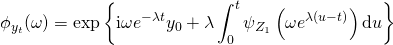as above. Let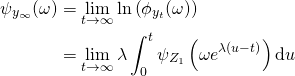be the cumulant generating function of the stationary distribution of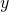. Then (not being fully rigorous)This relationship between the cumulant generating function of the stationary distribution of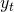and the cumulant generating function of the background driving Lévy process has been obtained by Barndorff-Nielsen (2001). Now, given that the stationary distribution ofiswith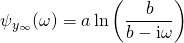we get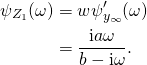As shown before, this is the cumulant generating function of a compound Poisson process with arrival rateand i.i.d. exponentially distributed increments with mean.

Integrated Time-Change

As shown in my previous post, the general solution for the characteristic function of the total activity process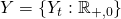is given by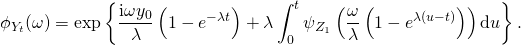The integral evaluates to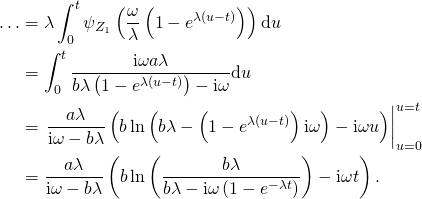Putting everything together, we getThis coincides with the expression given in Chapter 7.2 of Schoutens (2003).

References

Barndorff-Nielsen, Ole E. (2001) “Superposition of Ornstein-Uhlenbeck Type Processes,” Theory of Probability and its Applications, Vol. 45, No. 2, pp. 175-194

Barndorff-Nielsen, Ole E. and Neil Shephard (2001a) “Modelling by Lévy Processes for Financial Econometrics,” in Ole E. Barndorff-Nielsen, Thomas Mikosch and, and Sidney I. Resnick eds. Lévy Processes – Theory and Applications: Birkhauser, pp. 283-318

Cont, Rama and Peter Tankov (2004) Financial Modelling with Jump Processes: Chapman & Hall

Schotens, Wim (2003) Lévy Processes in Finance: John Wiley & Sons